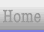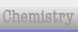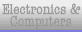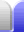#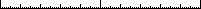### Length explained

Length is measured in metres (m).

Small lengths are measured in centimetres (cm), millimetres (mm) or even micrometres (µm) while long lengths (distances) might be measured in kilometres (km).

Objects do not have to be straight to have a length. A winding road has a length, even though it's not straight, and its length is greater than a direct path between the start and end points.The circumference of a circle also has a length - it just happens to be curved in the shape of a circle. Its length is a fixed multiple of the diameter of the circle. This multiple, or ratio, is called pi (a letter of the Greek alphabet), with the symbol π (which is the Greek letter).

π = C/d

π = 3.14159 26535 89793 23846 26433 83279 50288 41971 69399 37510... (50dp)

Whatever size the circle is and however big the diameter is, the circumference will always be pi times the diameter, so if the diameter of a circle is one metre, the circumference will be pi metres.

### Calculating Length

Straight objects have their length found by simply measuring the object. However, there are some calculations that can be done.

#### Length of a circle circumference:

π x diameter
π·d#### Length of a rectangle edge (eg, picture frame):

2 x (length + height)

### Prefixes

1,000 µm = 1 mm
1,000 mm = 1 m
1,000 m = 1 km

10 mm = 1 cm
10 cm = 1 dm
10 dm = 1 m
100 cm = 1 m

1 ly = 9,460,730,472,580.8 km (about 9.461 Pm)
1 ly = 63,241 AU

For more on prefixes see the Units & Prefixes page.

Particularly long distances might be measured in astronomical units (AU, the distance from the Sun to Earth, which is about 150 million kilometers) or light years - the distance light travels in a year (moving at about 300 million metres per second).

Shorter astronomical distances can actually be measured in light seconds, for example light takes about a second and a half to travel from Earth to Moon, as shown in this animation (which for some reason takes over 3 seconds to play in my browser):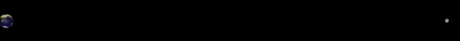### Random facts – more on pi

A value of pi truncated to 11 decimal places is accurate enough to calculate the circumference of Earth with a precision of a millimeter.

The Feynman Point is the sequence of six 9s which begins at the 762nd decimal place of π. It is named after physicist Richard Feynman, who once stated during a lecture he would like to memorize the digits of π until that point, so he could recite them and quip "nine nine nine nine nine nine and so on." The humorous irony of this statement is the suggestion that π is in fact rational, since this is falsely implied by an apparently infinitely recurring sequence of 9s.

π = 3. 1415926535897 9323846264338 3279502884197 1693993751058 2097494459230 7816406286208 9986280348253 4211706798214 8086513282306 6470938446095 5058223172535 9408128481117 4502841027019 3852110555964 4622948954930 3819644288109 7566593344612 8475648233786 7831652712019 0914564856692 3460348610454 3266482133936 0726024914127 3724587006606 3155881748815 2092096282925 4091715364367 8925903600113 3053054882046 6521384146951 9415116094330 5727036575959 1953092186117 3819326117931 0511854807446 2379962749567 3518857527248 9122793818301 1949129833673 3624406566430 8602139494639 5224737190702 1798609437027 7053921717629 3176752384674 8184676694051 3200056812714 5263560827785 7713427577896 0917363717872 1468440901224 9534301465495 8537105079227 9689258923542 0199561121290 2196086403441 8159813629774 7713099605187 0721134999999 ...Home Astronomy Chemistry Electronics & Computers Mathematics Physics Field Trips Turn on javascript for email link